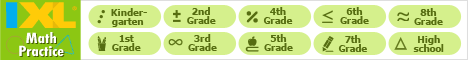Java Games: Flashcards, matching, concentration, and word search.# Triangle Vocabulary

AB
Triangle,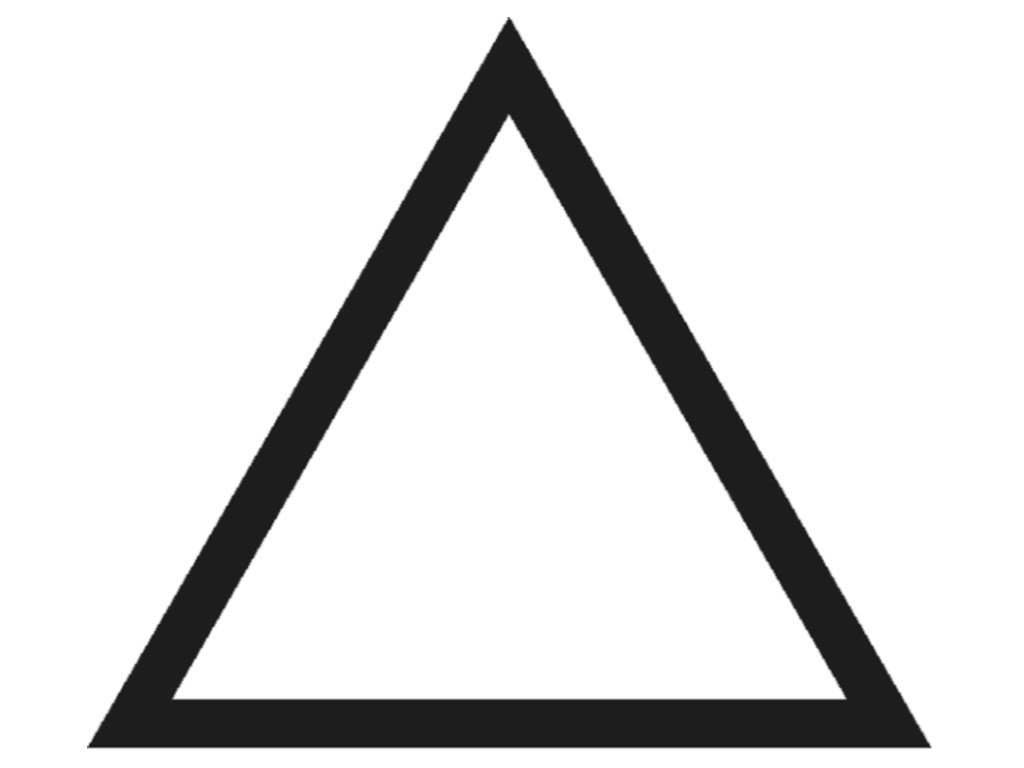3 sides, 3 angles
180°3 angles in triangle always add up to this
Straight angleIs always 180°
Equilateral triangle,3 equal sides, 3 equal angles, always 60°
Isosceles triangle,2 equal sides, 2 equal angles
Scalene triangle,No equal sides, no equal angles
Acute triangle,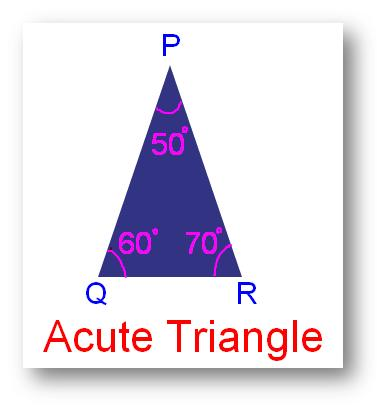All angles are less than 90°
Right triangle,Has a right angle (90°)
Obtuse triangle,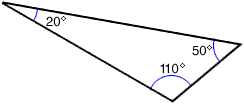Has an angle greater than 90°
Right scalene triangle,Has a right angle (90°) and no equal sides or angles
Right isosceles triangleHas a right angle (90°) and 2 equal angles (45°) and 2 equal sides
Obtuse scalene triangle,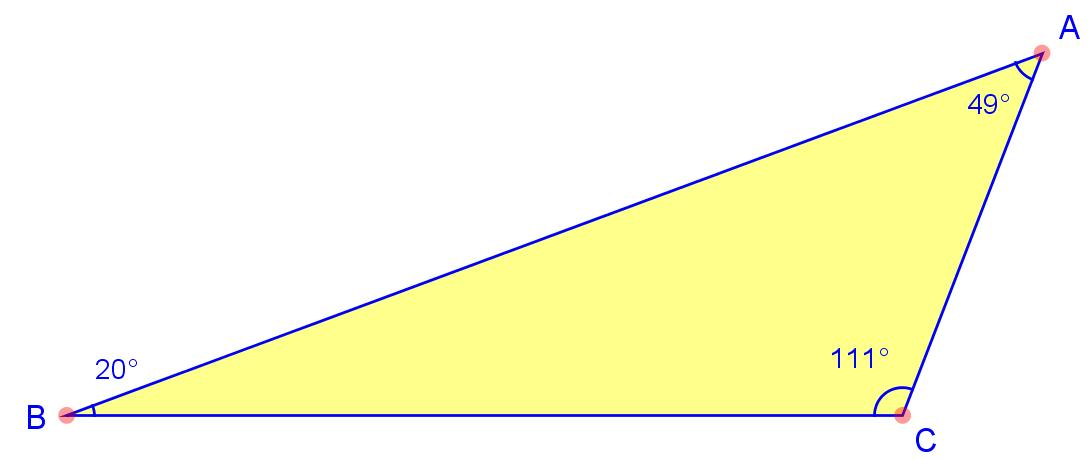Has an angle <90° and no equal sides or angles
Obtuse isosceles triangle,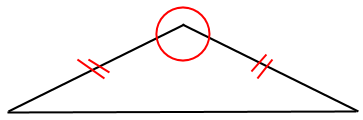Has an angle >90° and two equal sides
Acute scalene triangle,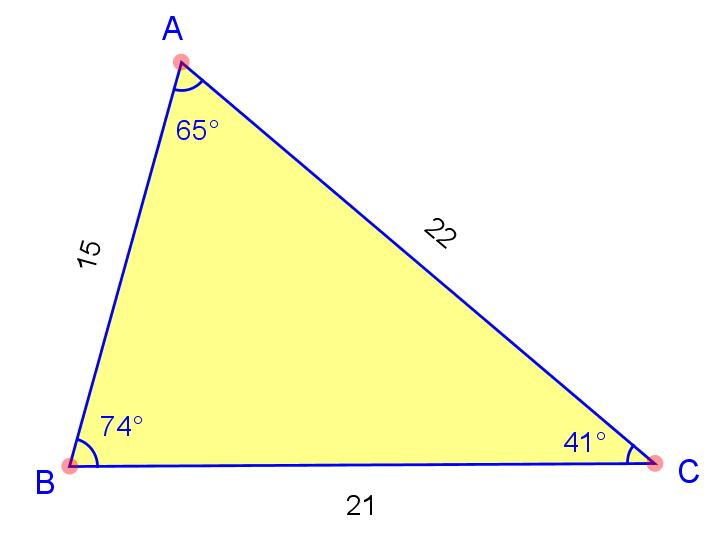Has all angles <90° and no equal sides or angles
Acute isosceles triangle,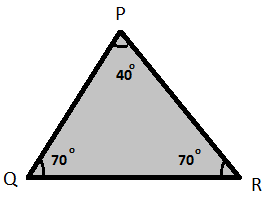Has all angles <90° and two equal sides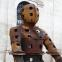Ms. Finley The Village School Houston, TX

 This activity was created by a Quia Web subscriber. Learn more about QuiaCreate your own activities